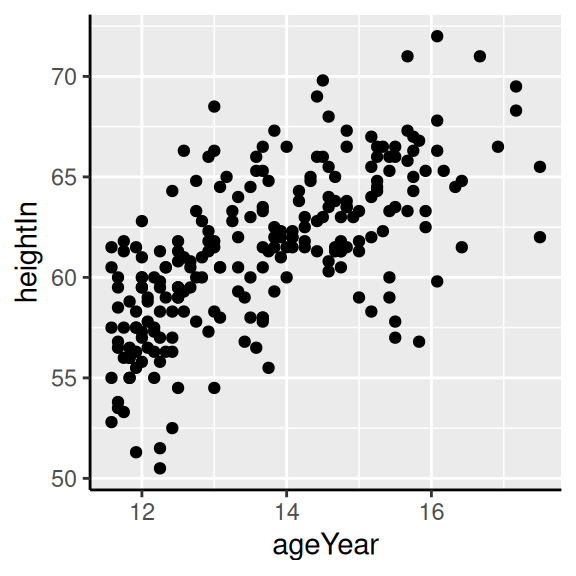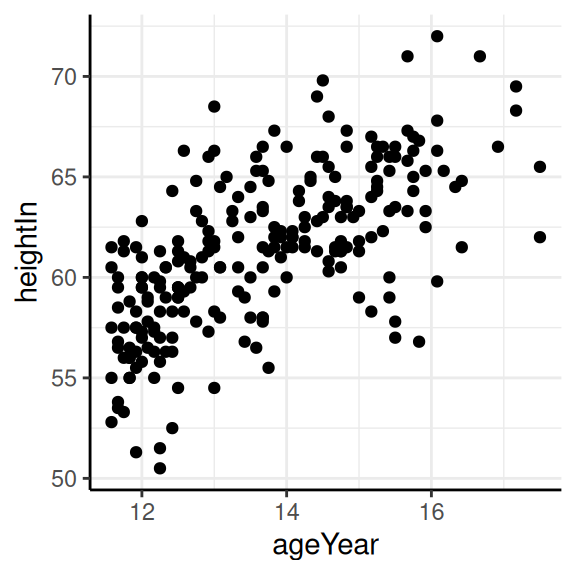## 8.13 Showing Lines Along the Axes

### 8.13.1 Problem

You want to display lines along the x- and y-axes, but not on the other sides of the graph.

### 8.13.2 Solution

Using themes, use `axis.line` (Figure 8.24):

``````library(gcookbook)  # Load gcookbook for the heightweight data set

hw_plot <- ggplot(heightweight, aes(x = ageYear, y = heightIn)) +
geom_point()

hw_plot +
theme(axis.line = element_line(colour = "black"))``````

### 8.13.3 Discussion

If you are starting with a theme that has a border around the plotting area, like theme_bw(), you will also need to unset panel.border (Figure 8.24, right):

``````hw_plot +
theme_bw() +
theme(panel.border = element_blank(), axis.line = element_line(colour = "black"))``````Figure 8.24: Scatter plot with axis lines (left); With `theme_bw()`, `panel.border` must also be made blank (right)

If the lines are thick, the ends will only partially overlap (Figure 8.25, left). To make them fully overlap (Figure 8.25, right), set `lineend = "square"`:

``````# With thick lines, only half overlaps
hw_plot +
theme_bw() +
theme(
panel.border = element_blank(),
axis.line = element_line(colour = "black", size = 4)
)
#> Warning: The `size` argument of `element_line()` is deprecated as of ggplot2 3.4.0.
#> This warning is displayed once every 8 hours.
#> Call `lifecycle::last_lifecycle_warnings()` to see where this warning was
#> generated.

# Full overlap
hw_plot +
theme_bw() +
theme(
panel.border = element_blank(),
axis.line = element_line(colour = "black", size = 4, lineend = "square")
)``````
<img src=“R-Graphics-Cookbook-2e_files/figure-html/FIG-AXES-AXIS-LINE-LINEEND-1.png” alt=“With thick lines, the ends don’t fully overlap (left); Full overlap with `lineend="square"` (right)” width=“288” /><img src=“R-Graphics-Cookbook-2e_files/figure-html/FIG-AXES-AXIS-LINE-LINEEND-2.png” alt=“With thick lines, the ends don’t fully overlap (left); Full overlap with `lineend="square"` (right)” width=“288” />

Figure 8.25: With thick lines, the ends don’t fully overlap (left); Full overlap with `lineend="square"` (right)